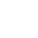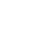LV. 50
GP 8k^^^^^^^^^^^^^^^^^^^^^^^^^^^^^^

^^^^^^^^^^^^^^^^^^^^^^^^^^^^^^^^

*原文作「日耳曼」，但這個可以當作德國的稱謂，所以改為使用共同源流的「條頓」更方便區分。

^^^^^^^^^^^^^^^^^^^^^^^^^^^^^

^^^^^^^^^^^^^^^^^^^^^^^^^^^

face基於日前微軟官方表示 Internet Explorer 不再支援新的網路標準，可能無法使用新的應用程式來呈現網站內容，在瀏覽器支援度及網站安全性的雙重考量下，為了讓巴友們有更好的使用體驗，巴哈姆特即將於 2019年9月2日 停止支援 Internet Explorer 瀏覽器的頁面呈現和功能。